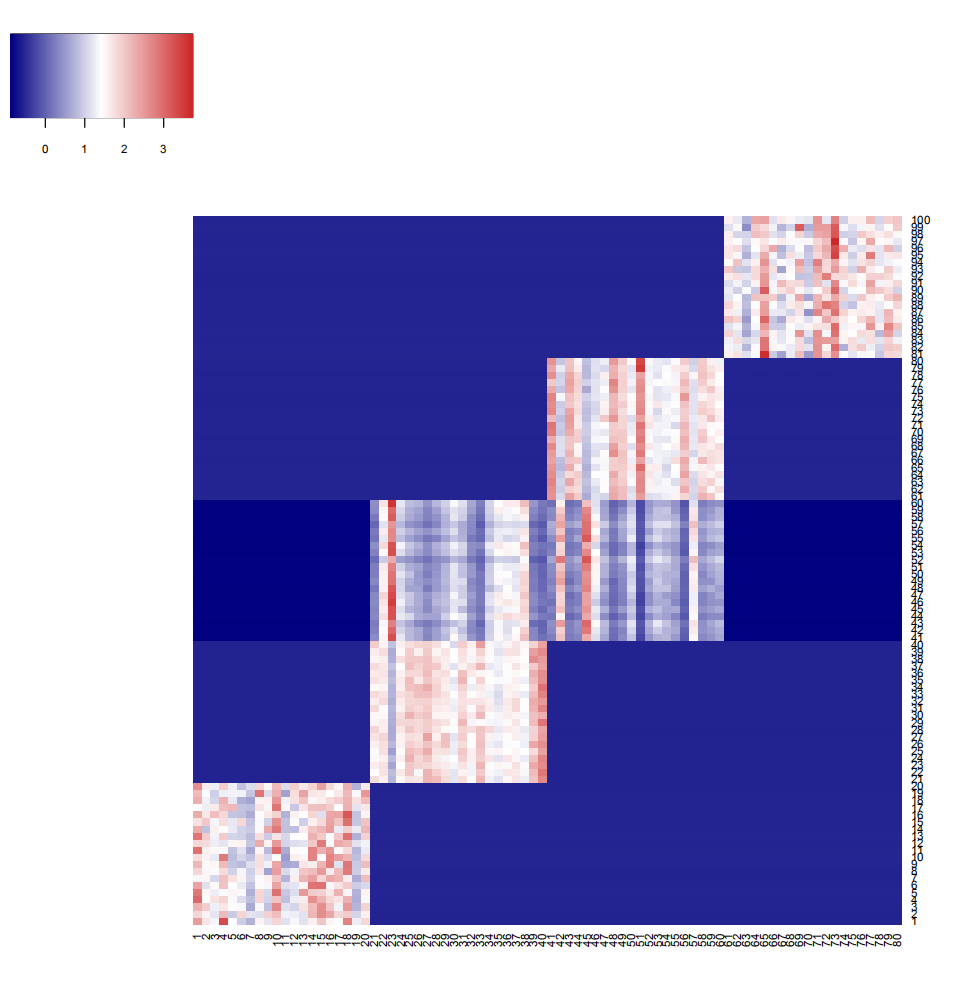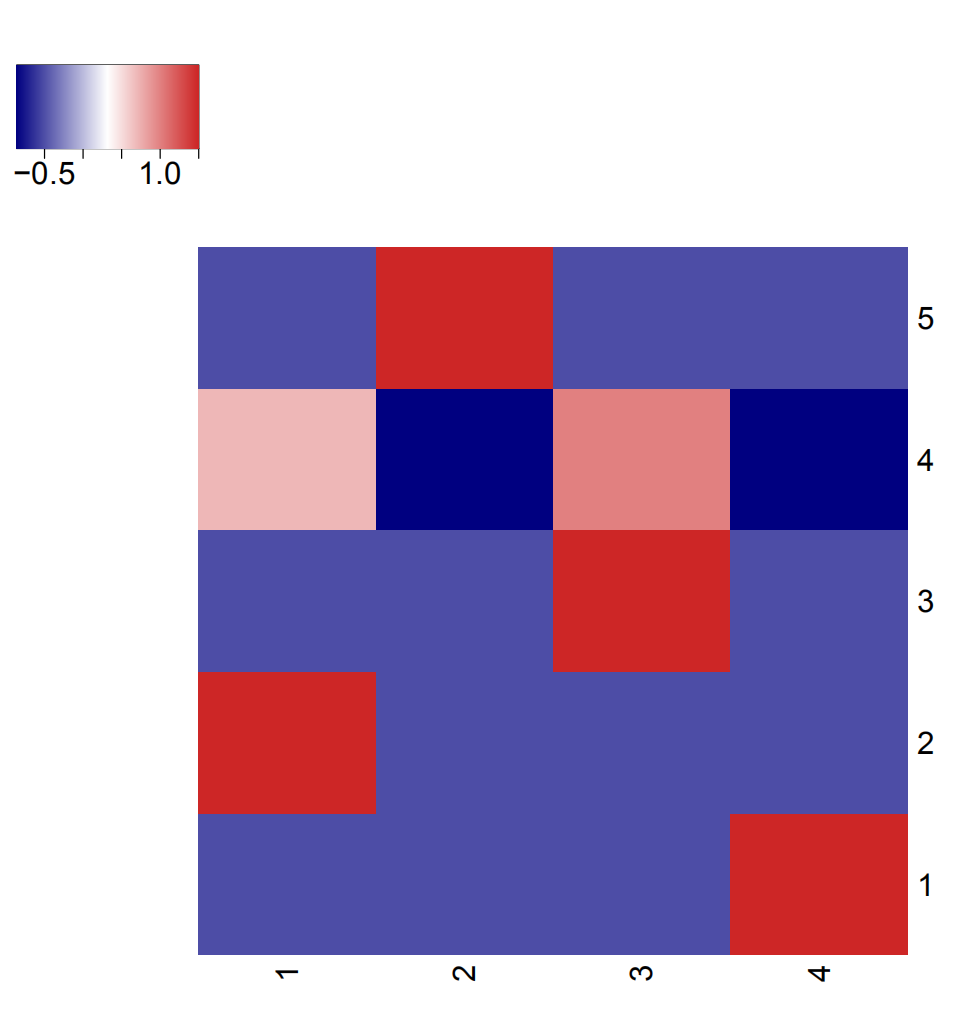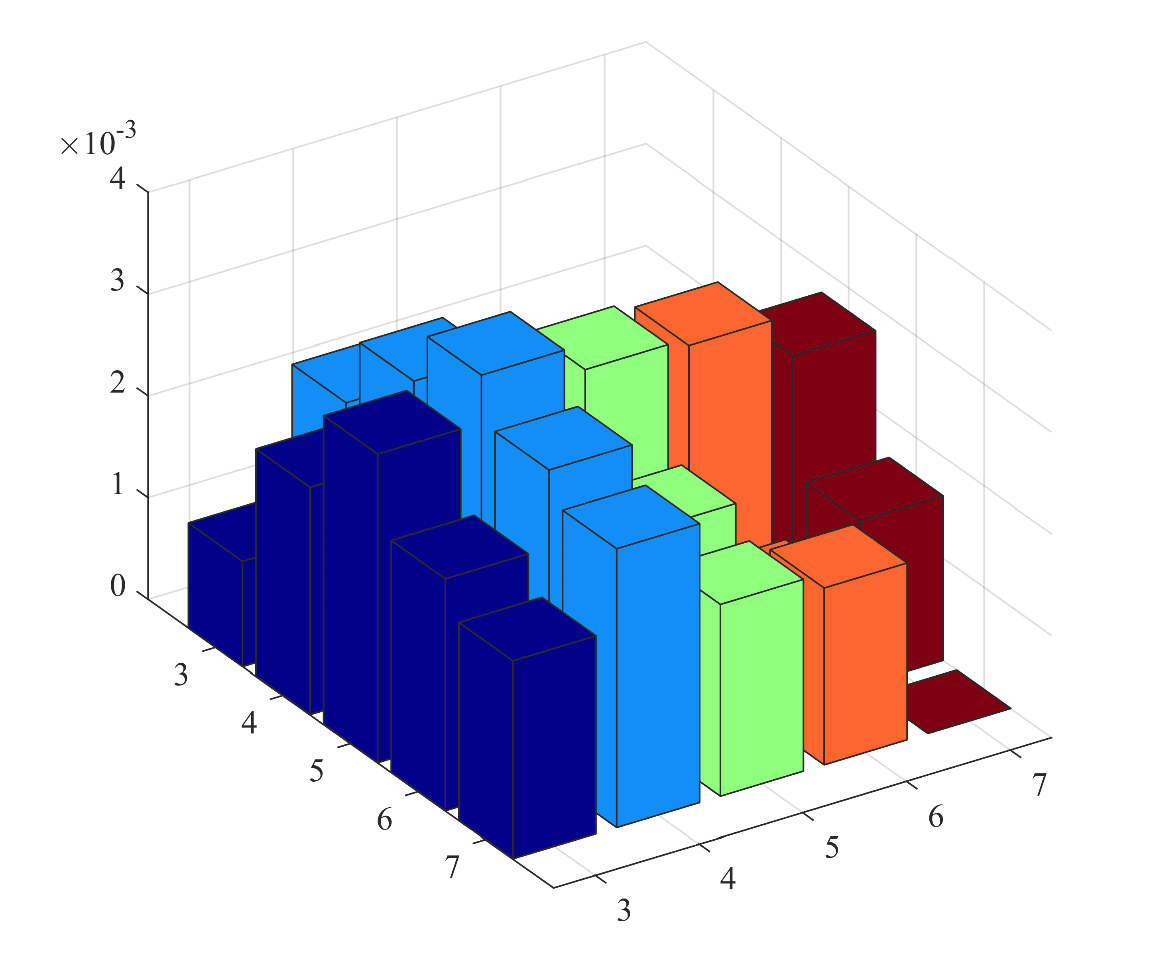An Introduction to the package OSNMTF

2019-11-24

This vignette presents the OSNMTF,which implements a noval framework named orthogonal sparse non-negative matrix tri-factorization (OSNMTF) to conduct bi-clustering in R. The objective is to provide an implementation of the proposed method, which is designed to obtain cancer subtyping, gene set functional enrichemnt and subtype specific drug target identification. It was achived by factorizing the data matrix (e.g. mRNA data with each row as a sample) into the row coefficient matrix, the association matrix and the column coefficient matrix. Orthogonal constraints was introduced to improve the interpretability and rank the importance of genes. Sparsity constraint was introduced to meet the prior knowledge that each cancer subtype should be related to only a few gene sets.

Installation

The latest stable version of the package can be installed from any CRAN repository mirror:

#Install
install.packages('OSNMTF')
library(OSNMTF)

The latest development version is available from https://cran.r-project.org/package=OSNMTF and may be downloaded from there and installed manually:

install.packages('/path/to/file/OSNMTF.tar.gz',repos=NULL,type="source")

Support: Users interested in this package are encouraged to email to Xiaoyao Yin (yinxy1992@sina.com) for enquiries, bug reports, feature requests, suggestions or OSNMTF-related discussions.

Usage

We will give an example of how to use this packge hereafter.

Simulation data generation

We generate simulated data with five row clusters and four column clusters via the function simu_data_generation. The simulated data matrix Sim is a similarity matrix of two group of samples X1 and X2. The first group of samples X1 is comprised of 100 samples with 100 features, belonging to 5 clusters, and each cluster consists of 20 samples with mean {10,20,30,40,50} and variance 1. The second group of samples X2 is comprised of 80 samples with 100 features, belonging to 4 clusters, and each cluster consists of 20 samples with mean {5,10,15,20,25} and variance 1. The data can be generated by running:

simu_data = simu_data_generation()

Structure of the simulated data: The simulation data has clear data structure, as shown in the Heatmap:OSNMTF: Factorize the simulation data into the row coefficient matrix, the association matrix and the column coefficient matrix with orthogonal and sparsity constraints.

# Factorize the matrix with OSNMTF
OSNMTF_res <- OSNMTF(simu_data,k=5,l=4)
# Get the row coefficient matrix
row_coef <- OSNMTF_res[][]
# Get the association matrix
asso_matrix <- OSNMTF_res[][]
# Get the column coefficient matrix
column_coef <- OSNMTF_res[][]
# Get the row cluster results
row_cluster <- OSNMTF_res[][]
# Get the column cluster results
column_cluster <- OSNMTF_res[][]

Structure of the association matrix: The association matrix has the same data structure as the simulation data, as shown in the Heatmap:Cluster number evaluation: Evaluate the proper row and column cluster number with the metric average residue.

# Specify your desired cluster number evaluation interval by your prior knowledge of the data
ASR_matrix <- matrix(0,5,5)
for (i in 1:5)
{
rankk <- i+2
for (j in 1:5)
{
rankl <- j+2
temp_res <- OSNMTF(simu_data,k=rankk,l=rankl)
row_clu1 <- temp_res[][]
col_clu1 <- temp_res[][]
# MNSR_matrix[i,j] <- MNSR(row_clu1,col_clu1,simi_matr1)
ASR_matrix[i,j] <- ASR(row_clu1,col_clu1,simu_data)
}
}

Results of the average residue: The average residue is shown in the 3D Histgram:The larger the value, the better the corresponding cluster number. It can be found that (5,4) is the best with regard to the average residue metric, which is consistent with our data structure.